Courses
Courses for Kids
Free study material
Offline Centres
MoreLast updated date: 06th Dec 2023
Total views: 282.9k
Views today: 6.82k

# Which one of the following is not expected to undergo iodoform reaction $?$ (A) Propan $- 2 -$ ol(B) $1 -$ Phenyl ethanol(C) $2 -$ Butanol(D) Ethanol(E) Diphenyl methanolVerified
282.9k+ views
Hint :Iodoform is an iodine containing organic compound with molecular formula $CH{I_3}$ . It is a crystalline solid that has a characteristic pale yellow colour and distinct odour. Iodoform test is used to determine the presence of $C{H_3}CO$ or $C{H_3}CH(OH)$ groups in the given organic compound. It is a test given by certain aldehydes, ketones, and secondary alcohols.

Iodoform test is given by aldehydes, ketones, secondary alcohols that have an alpha methyl group attached to the carbon containing functional group. It tests the presence of $C{H_3}CO$ and $C{H_3}CH(OH)$ . When iodine reacts with a base (NaOH) and the carbonyl group of the organic compound, it forms a yellow precipitate which is iodoform. Below is an example of an iodoform test.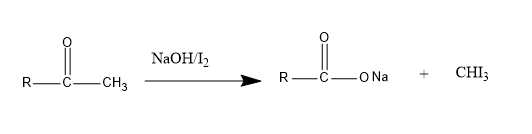Now, let us examine the given compounds.
(A)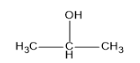It is a secondary alcohol and undergoes iodoform test since it contains $C{H_3}CH(OH)$ group.
(B)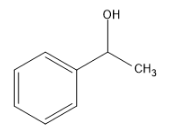Compound contains the $C{H_3}CH(OH)$ group and therefore gives positive results for the iodoform test.
(C)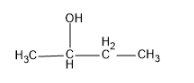$2 -$ Butanol also contains $C{H_3}CH(OH)$ group and hence a yellow precipitate of iodoform is formed.
(D)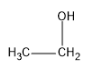Ethanol is the only primary alcohol that gives an iodoform test.
(E)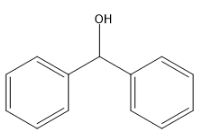It does not contain any alpha methyl group. Iodoform is not formed when the given compound reacts with NaOH and ${I_2}$ . There is no $C{H_3}CO$ or $C{H_3}CH(OH)$ group.
Therefore, the right option is (E) Diphenyl methanol.

Note :
Iodoform also known as triiodomethane or carbon triiodide has a distinct odour that is similar to chloroform. It has antimicrobial properties and is used as disinfectant. It is also used as an antiseptic.
Acetaldehyde is the only aldehyde that gives positive iodoform test.
Iodoform test is an important reaction used for the production of iodoform.